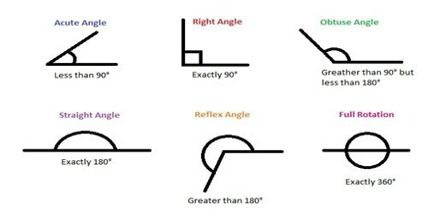Mathematic

# Lines and AnglesThe aim of this lecture is to present on Lines and Angles. Line together with point, is a basic concept of elementary geometry. The idea of line is an abstraction that distills our intuition that a straight line is the shortest way between two points. However, we distinguish between a line and a line segment. A line segment includes the endpoints, i.e. the points that it joins. An angle is a combination of two rays with a common endpoint. The latter is known as the vertex of the angle and the rays as the sides, sometimes as the legs and sometimes the arms of the angle.